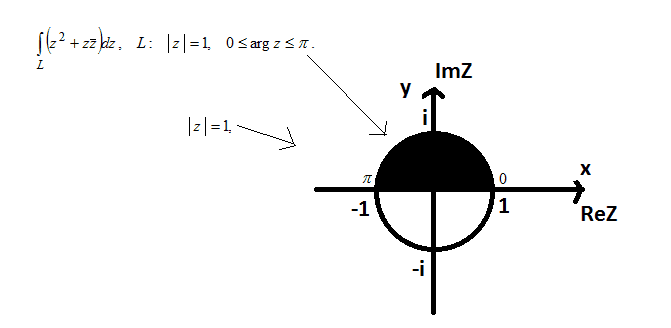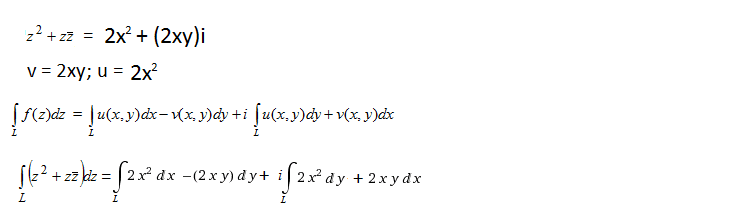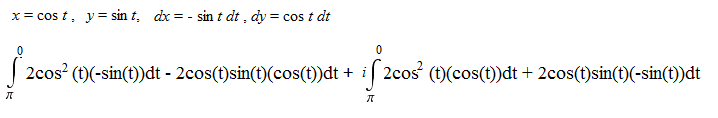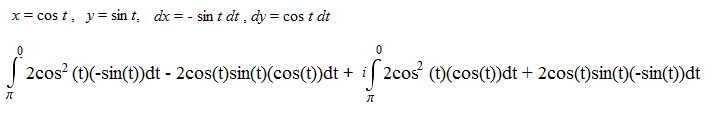# Calculate complex integral as line integral

evol_w10lv

## Homework Statement

We need to calculate this complex integral as line integral:## The Attempt at a Solution

This is correct, I guess:Are dx, dy, x, y chages correct or there is other method to use?

jackmell

## Homework Statement

We need to calculate this complex integral as line integral:## The Attempt at a Solution

This is correct, I guess:Are dx, dy, x, y chages correct or there is other method to use?

Not hard to numerically integrate it and check your results right?

Code:
NIntegrate[(z^2 + z*Conjugate[z])*I*Exp[I*t] /. z -> Exp[I*t], {t, Pi, 0}]

but I just let $z=e^{it}$ to check that. What's wrong with just doing that symbolically from the start.
So my suggestion is for you to compute the expression your derived, compute it numerically in Mathematica or other, and thirdly, by letting $z=e^{it}$ and computing it by evaluating the integral in t and then compare the three.

evol_w10lv
Of course... thanks for idea. I cheked results and they were equal.